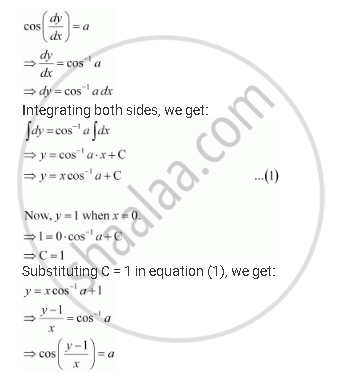Share

# For Each of the Differential Equations Find a Particular Solution Satisfying the Given Condition: Cos (Dx/Dy) = A(A in R); Y = 1 When X = 0 - CBSE (Commerce) Class 12 - Mathematics

ConceptMethods of Solving First Order, First Degree Differential Equations Differential Equations with Variables Separable

#### Question

For each of the differential equations find a particular solution satisfying the given condition:

cos (dx/dy) = a(a in R); y = 1 " when "x = 0

#### SolutionIs there an error in this question or solution?

#### APPEARS IN

NCERT Solution for Mathematics Textbook for Class 12 (2018 to Current)
Chapter 9: Differential Equations
Q: 13 | Page no. 396
Solution For Each of the Differential Equations Find a Particular Solution Satisfying the Given Condition: Cos (Dx/Dy) = A(A in R); Y = 1 When X = 0 Concept: Methods of Solving First Order, First Degree Differential Equations - Differential Equations with Variables Separable.
S